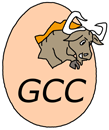# g++

Implementation of programming language C++

g++ is the short nickname for GNU C++ compiler, which is included in GNU Compiler Collection.

The Collection was extended to handle C++ in December 1987, the same year as it was released. It is distributed under GNU GPL and is an important example of free software.GCC logo

## Examples:

### Hello, World!:

Example for versions Borland C++ Builder 6, Microsoft Visual C++ 6, Microsoft Visual C++ 9 (2008), g++ 3.4.5
``````#include <iostream>

int main(void)
{
std::cout << "Hello, World!" << std::endl;
return 0;
}
``````

### Factorial:

Example for versions Borland C++ Builder 6, Microsoft Visual C++ 6, Microsoft Visual C++ 9 (2008), g++ 3.4.5

This example uses recursive factorial definition.

``````#include "stdio.h"

__int64 factorial(__int64 n)
{
return ( n==0 ? 1 : n*factorial(n-1) );
}

int main(int argc, char* argv[])
{
for (int n=0; n<=16; n++)
printf( "%d! = %I64d\n", n, factorial(n) );
return 0;
}
``````

### Hello, World!:

Example for versions Borland C++ Builder 6, Turbo C++ 1.01, g++ 3.4.5, gcc 3.4.5, gcc 3.4.5 (Objective-C), gcc 4.2.4, tcc 0.9.25
``````#include <stdio.h>

int main()
{
printf("Hello, World!\n");
return 0;
}
``````

### Factorial:

Example for versions Borland C++ Builder 6, g++ 3.4.5

This example uses recursive factorial definition.

``````#include <iostream>

unsigned long long factorial(unsigned long long n)
{
if (n == 0)
return 1;
else
return n * factorial (n - 1);
}

int main(void)
{
for (int n = 0; n <= 16; n++)
std::cout << n << "! = " << factorial(n) << std::endl;
return 0;
}
``````

### Fibonacci numbers:

Example for versions Borland C++ Builder 6, Microsoft Visual C++ 6, Microsoft Visual C++ 9 (2008), g++ 3.4.5

This example uses recursive definition of Fibonacci numbers.

``````#include <iostream>

int fibonacci(int n)
{
return ( n<=2 ? 1 : fibonacci(n-1) + fibonacci(n-2) );
}

int main(void)
{
for (int n=1; n<=16; n++)
std::cout << fibonacci(n) << ", ";
std::cout << "..." << std::endl;
return 0;
}
``````

Example for versions Borland C++ Builder 6, Microsoft Visual C++ 9 (2008), g++ 3.4.5, gcc 3.4.5, gcc 3.4.5 (Objective-C), gcc 4.2.4, tcc 0.9.25

This example works both for C and C++, as well as for Objective-C which is superset of C.

``````#include <math.h>
#include <stdio.h>

int main()
{
int A, B, C;
double D;
printf("A = ");
scanf("%d", &A);
if (A == 0) {
return 0;
}

printf("B = ");
scanf("%d", &B);
printf("C = ");
scanf("%d", &C);

D = B * B - 4 * A * C;
if (D == 0) {
printf("x = %f\n", -B / 2.0 / A);
return 0;
}

if (D > 0) {
printf("x1 = %f\nx2 = %f\n",
(-B + sqrt(D)) / 2.0 / A, (-B - sqrt(D))/ 2.0 / A);
} else {
printf("x1 = (%f, %f)\nx2 = (%f, %f)\n",
-B / 2.0 / A, sqrt(-D) / 2.0 / A, -B / 2.0 / A, -sqrt(-D) / 2.0 /A);
}
return 0;
}
``````

### CamelCase:

Example for versions Borland C++ Builder 6, Microsoft Visual C++ 9 (2008), g++ 3.4.5

This example is based on character-by-character string processing.

`getline` reads a string (delimited with end of line) from argument stream. Function `tolower` works only with single characters, so to convert whole string to lower case it is used with `transform` function. The latter applies `tolower` to all elements in the range `[text.begin(), text.end())` and stores the results in a range starting with `text.begin()` again.

After this the string is processed char-by-char. Each character is checked for being alphabetic; if it is, it is appended to the resulting string (converted to upper case if previous character was non-alphabetic); if it is not, it only affects `lastSpace` (which is true only if last character was non-alphabetic).

`isalpha` works with both uppercase and lowercase letters, so it was possible not to convert the input string to lowercase, but rather to convert each appended character.

``````#include <string>
#include <iostream>
#include <algorithm>

using namespace std;

int main() {
string text, cc="";
bool lastSpace = true;
getline(cin, text);
transform(text.begin(), text.end(), text.begin(), (int (*)(int))tolower);
for (int i=0; i<text.size(); i++)
if (isalpha(text[i])) {
if (lastSpace)
cc += toupper(text[i]);
else
cc += text[i];
lastSpace = false;
}
else {
lastSpace = true;
}
cout << cc << endl;
return 0;
}
``````